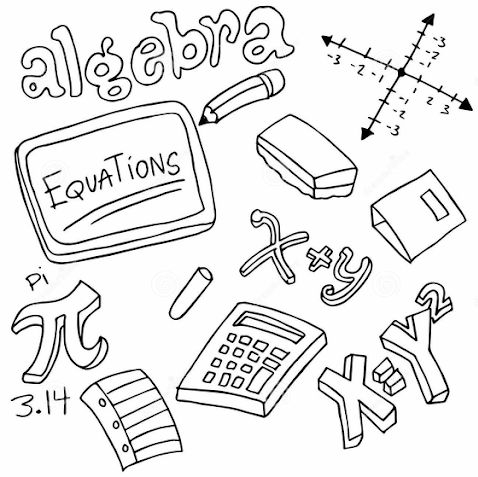# Algebraic Expressions

Welcome to Class !!

In today’s Mathematics class, We will be discussing Algebraic Expressions. We hope you enjoy the class!

Content

• Definition with examples
• Expansion of algebraic expression
• Factorization of simple algebraic expressionsDefinition with examples

In algebra, letters stand for numbers. The numbers can be whole or fractional, positive or negative.

Example

Simplify the following

• -5 x 2y
• -3a x -6b
• -14a/7
• -1/3 of 36x2

Solution

1)    -5 x 2y = -5 x (+2) x y

= -(5 x 2) x y = -10y

2)    -3a x -6b = (-3) x a (-6) x b

= (-3) x (-6) x a x b = 18ab

3)

4)

-1/3 of 36x2 = (+36) x x2 = – (36/3) x x2

(-3)

= -12x2

Self Evaluation

Simplify the following

1. -16x/8
2. (-1/10) of 100z
3. (-2x) x (-9y)

Removing brackets

Example

Remove brackets from the following

a. 8 (2c + 3d)    (b) 4y (3x-5)   (c) (7a-2b) 3a

Solution

(a) 8(2c+3d) = 8 x 2c + 8 x 3d

= 16c + 24d

(b) 4y(3x-5) = 4y x 3x – 4y x 5

= 12xy – 20y

(c) (7a-2b)3a = 7a x 3a – 2b x 3a

=21a2 – 6ab

Self Evaluation

Remove brackets from the following

1. -5x(11x – 2y)
2.  -p(p – 5q)
3. (2c + 8d)(-2)

##### Expanding algebraic expressionsThe expression (a+2) (b-5) means (a+2) x (b-5)

The terms in the first bracket, (a+2), multiply each term in the second bracket, b-5.

Example

Expand the following

1. (a+b) (c+d)
2. (6-x) (3+y)
3. (2p-3q) (5p-4)

Solution

(1) (a+b)(c+d) = c(a+b) + d(a+b)

= ac + bc + ad + bd

(2) (6-x)(3+y) = 3(6-x) + y (6-x)

= 18 -3x +6y – xy

(3) (2p-3q)(5p-4) = 5p(2p – 3q)-4(2p-3q)

= 10p2 – 15pq – 8p + 12q

Self Evaluation

Expand the following

(a) (3+d)(2+d)

(b) (3x+4)(x-2)

(c) (2h-k)(3h+2k)

(d) (7m-5n)(5m+3n)

### Factorization of algebraic expression

Example:

Factorize the following

(a) 12y + 8z

(b) 4n2 – 2n

(c) 24pq – 16p2

Solution

(a) 12y +8z

The HCF of 12y and 8z is 4

12y +8z =

= 4(3y + 2z)

(b) 4n2 – 2n

The HCF of 4n2 and 2n is 2n

4n2 – 2n =

= 2n (2n-1)

(c) 24pq – 16p2

The HCF of 24pq and 16p2 is 8p

24pq – 16p2

= 8p(3q – 2p)

Self Evaluation

Factorize the following:

1. 2abx + 7acx (b) 3d2e + 5d2
2. 12ax + 8bx

New General Mathematics, UBE Edition, Chapter 1, pages 20-21

Essential Mathematics by A J S Oluwasanmi, Chapter 1, pages 1-4

WEEKEND ASSIGNMENT

1. Simplify (-6x) x (-x) =_____ (A) 6x (b) 6x2 (C) -6x (D) -6x2
2. Remove brackets from -3(12a – 5)   (A) 15-36a (B) 15a-36 (C) 15a + 36 (C) 36a – 15
3. Expand (a+3)(a+4) (A) a2+7a+12 (B) a2+12a+7 (C) a2+12a-7 (D) a2+7a-12
4. Factorize abc + and  (A) ab(c+d)  (B) ac (b+d)  (C) ad(b+c) (D) abc(c+d)
5. Factorize 5a2 + 2ax  (A) a(5a+2x)  (B) 5(2a2+2x) (C) a(5x+2ax) (D) a2(5+2x)

THEORY

1. Expand the following:
1. (p+2q) (p+3q)
2. (5r+2s) (3r+4s)
1. Factorize the following
• -18fg – 12g
• -5xy + 10y

We have come to the end of this class. We do hope you enjoyed the class?

Should you have any further question, feel free to ask in the comment section below and trust us to respond as soon as possible.

In our next class, we will be talking about Algebraic Fractions. We are very much eager to meet you there.

Are you a Parent? Share your quick opinion and win free 2-month Premium Subscription

Don`t copy text!